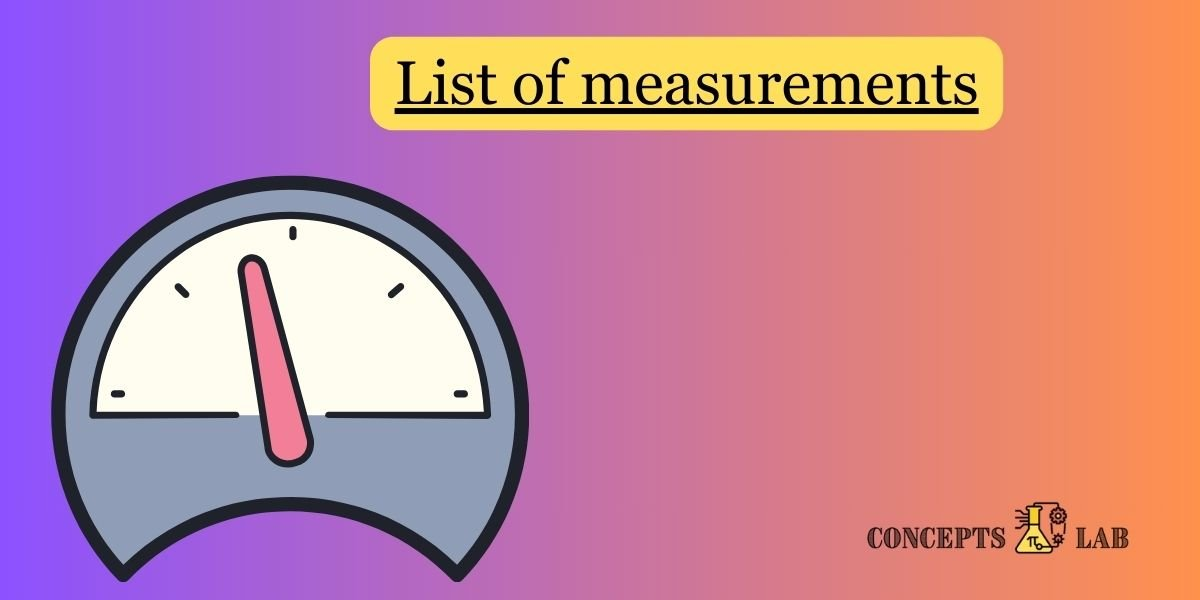Categories:

Here’s a list of measurements, their units, and common applications:

### Length:

• Unit: Meter (m)
• Applications:
• Measuring the height and width of objects.
• Determining distances between locations.
• Calculating the dimensions of a room or building.

### Mass:

• Unit: Kilogram (kg)
• Applications:
• Weighing objects.
• Measuring the mass of a person.
• Calculating the mass of substances in chemistry.

### Time:

• Unit: Second (s)
• Applications:
• Timing events.
• Measuring the duration of activities.
• Calculating speed and acceleration.

### Temperature:

• Unit: Celsius (°C) or Kelvin (K)
• Applications:
• Monitoring weather conditions.
• Controlling the temperature in cooking.
• Analyzing chemical reactions in laboratories.

### Electric Current:

• Unit: Ampere (A)
• Applications:
• Measuring the flow of electric charge.
• Calculating the power consumed by electrical devices.

### Luminous Intensity:

• Unit: Candela (cd)
• Applications:
• Describing the brightness of light sources.
• Standardizing light bulbs based on brightness.

### Amount of Substance:

• Unit: Mole (mol)
• Applications:
• Quantifying the number of particles (atoms, molecules) in a substance.
• Calculating concentrations in solutions.

### Area:

• Unit: Square meter (m²)
• Applications:
• Determining the size of a piece of land.
• Calculating the floor area of a room.

### Volume:

• Unit: Cubic meter (m³)
• Applications:
• Measuring the capacity of containers.
• Determining the volume of liquids or gases.

### Velocity:

• Unit: Meter per second (m/s)
• Applications:
• Describing the speed and direction of an object’s motion.
• Calculating the velocity of vehicles.

### Acceleration:

• Unit: Meter per second squared (m/s²)
• Applications:
• Describing changes in velocity.
• Analyzing motion in physics experiments.

### Pressure:

• Unit: Pascal (Pa)
• Applications:
• Measuring atmospheric pressure.
• Determining blood pressure in medicine.

### Frequency:

• Unit: Hertz (Hz)
• Applications:
• Describing the number of cycles per second in waves.
• Tuning musical instruments.

### Energy:

• Unit: Joule (J)
• Applications:
• Calculating the amount of energy consumed by appliances.
• Describing energy changes in physical processes.

### Power:

• Unit: Watt (W)
• Applications:
• Measuring the rate of energy transfer or consumption.
• Describing the output of electrical devices.

### Electric Charge:

• Unit: Coulomb (C)
• Applications:
• Quantifying the flow of electric charge in circuits.
• Measuring the charge on particles in physics experiments.

These units and measurements are fundamental in various scientific disciplines and everyday applications. Understanding and using them correctly is crucial for accurate scientific work and effective communication of quantitative information.